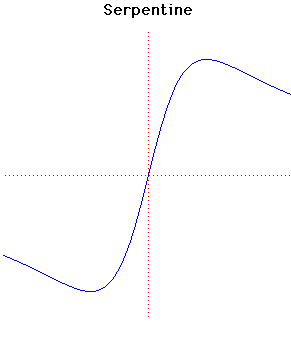# Curves

### SerpentineParametric Cartesian equation:
$x^{2}y + aby - a^{2}x = 0, ab > 0$

### Description

This curve was named and studied by Newton in 1701. It is contained in his classification of cubic curves which appears in Curves by Sir Isaac Newton in Lexicon Technicumby John Harris published in London in 1710. Harris's introduction to the article states:

The incomparable Sir Isaac Newton gives this following Ennumeration of Geometrical Lines of the Third or Cubick Order; in which you have an admirable account of many Species of Curves which exceed the Conick-Sections, for they go no higher than the Quadratick or Second Order.
[Here the older spelling has been preserved including conick, Quadratick and ennumeration].

Newton shows that the curve $f(x, y) = 0$, where $f(x, y)$ is a cubic, can be divided into one of four normal forms. The first of these is equations of the form
$xy^{2} + ey = ax^{3} + bx^{2} + cx + d$.
This is the hardest case in the classification and the serpentine is one of the subcases of this first normal form.

The serpentine had been studied earlier by de L'Hôpital and Huygens in 1692.

### Associated Curves

Definitions of the Associated curves